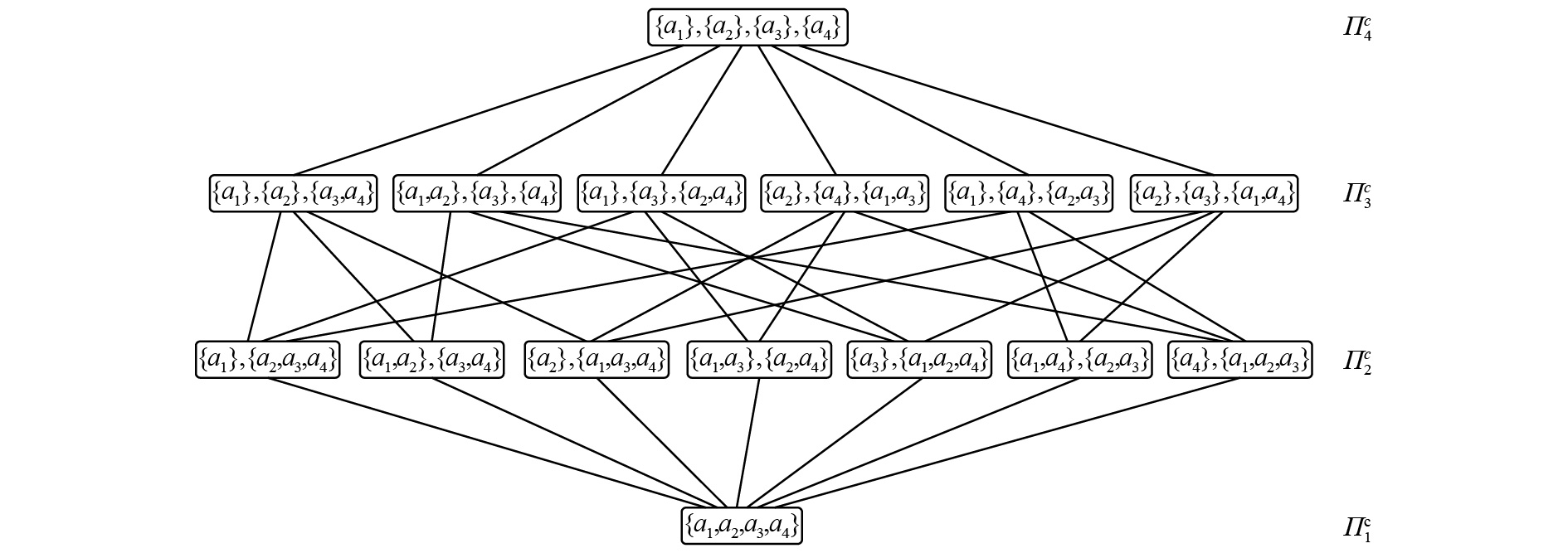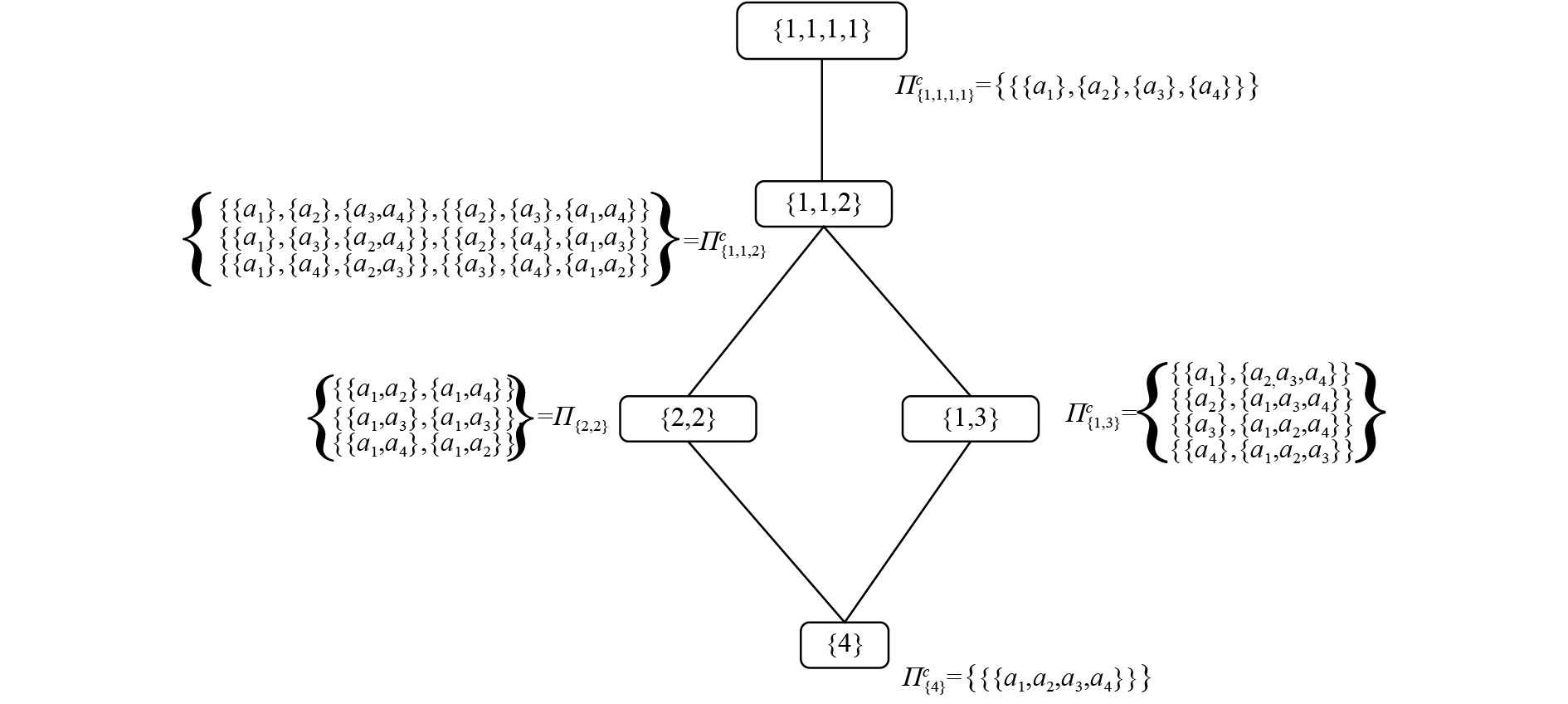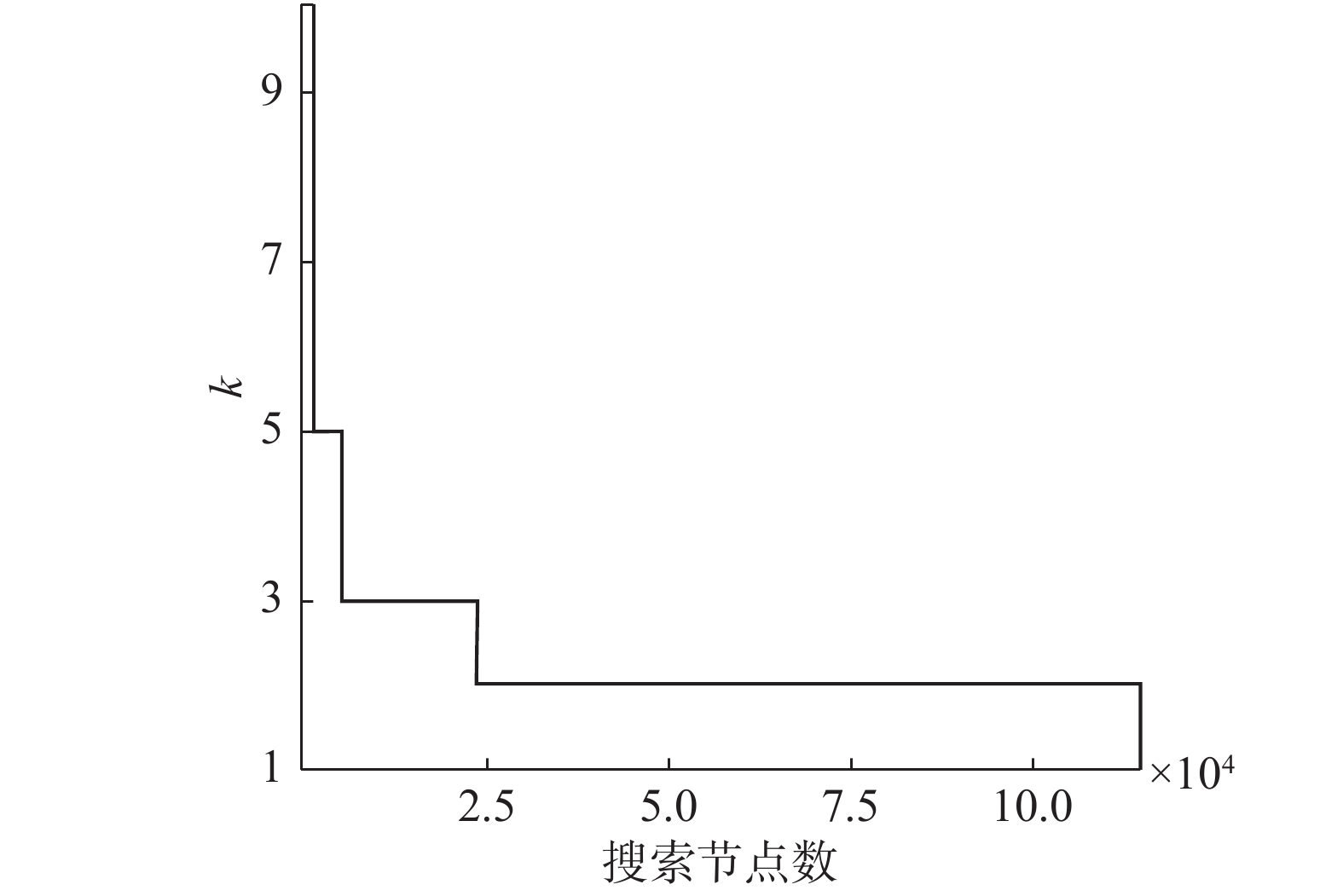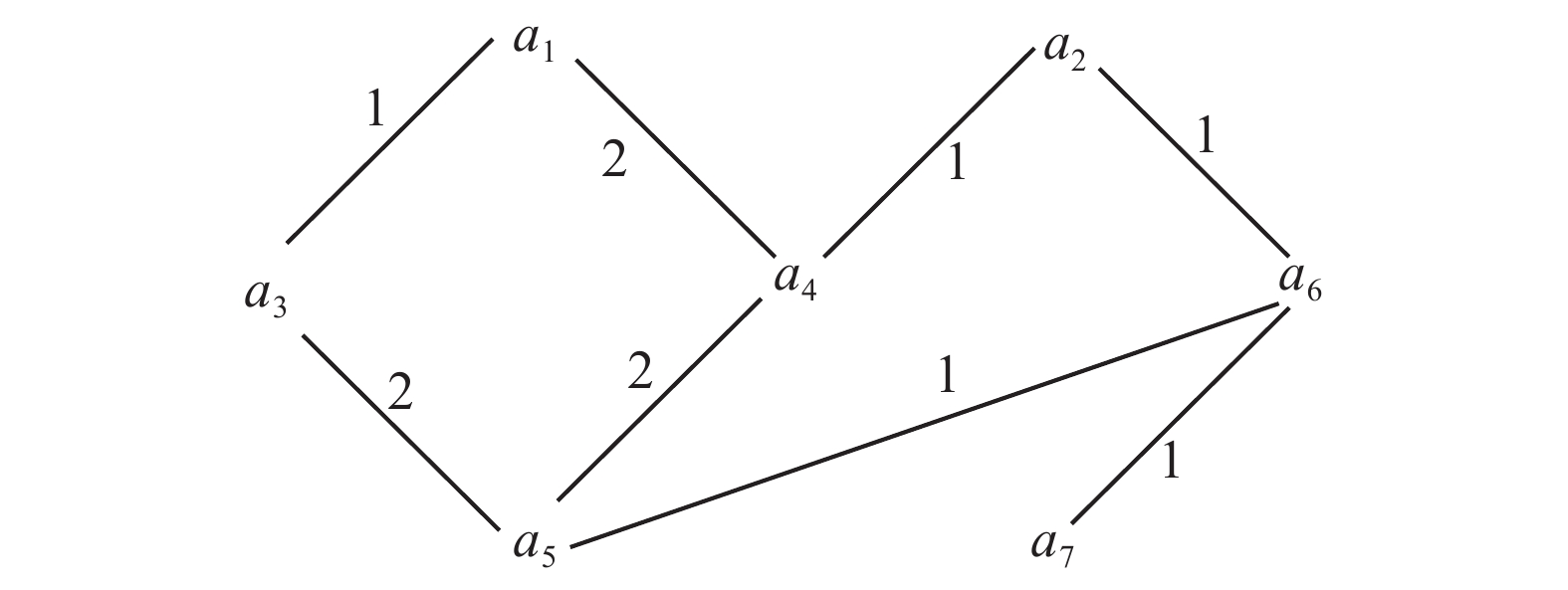﻿ 约束条件下联盟生成研究进展
«上一篇文章快速检索 高级检索

 智能系统学报2019, Vol. 14Issue (3): 413-422  DOI: 10.11992/tis.2018040540

### 引用本文REN Ziyi, TONG Xiangrong. Research progress of constrained coalition formation[J]. CAAI Transactions on Intelligent Systems, 2019, 14(3): 413-422. DOI: 10.11992/tis.201804054.### 文章历史

Research progress of constrained coalition formation
REN Ziyi , TONG XiangrongSchool of Computer and Control Engineering, Yantai University, Yantai 264005, China
Abstract: Coalition formation is one of the most important challenges in the research of multiagent systems. Currently, our main problem is how to divide Agent to maximize the social welfare. We assume that each Agent possesses the characteristics of rationality and self-interest to maximize its own interests. An Agent integrates with another Agent, which also maximizes the interest of the whole system. At present, the coalition formation problem presents notable computational challenges. If constraints are added during the coalition process, new algorithms are needed to solve the problem more rapidly and effectively. This paper mainly summarizes the study of coalition structure generation under constraint conditions. This paper comprises four parts: the coalition structure generation with the worst case guaranteed, the use of the dynamic programming to find the exact optimal solution, the near-optimal solution after formation of the coalition structure, and the optimal solution to the constrained coalition formation.
Key words: coalition structure    social welfare    coalition formation    constraint    characteristic function    coalition structure graph    coalition game    dynamic programming

Agent在联盟生成时达到最优方案不一定是全局最优解，但是应该是Nash最优解[6-7]或者次优解，因此联盟生成主要包括以下活动：

1) 联盟结构生成：Agent之间进行协商生成联盟，但不考虑各个联盟之间的协商。

2) 解决每个联盟优化问题：将Agent的任务和资源集中在一起，共同解决问题。Agent之间进行协商使联盟的社会福利尽可能最大化，即寻找最优的方法使得联盟本身的效用最大化。

3) 划分值：在进行收益分配的时候，常用的方法有两种，即公平(每个Agent所得到的收益是Agent在博弈中的贡献的体现)和稳定(存在没有和其他Agent协商而单独形成自己的自私利益联盟)。

1 基本定义 1.1 主要定义

 $V\left( {\rm CS} \right) = \sum\limits_{i = 1}^k {\nu ({C_i})}$

1.2 博弈类型

1) 特征函数博弈

2) 分区博弈

CFGs是PFGs的子集，联盟C在CFGs中有一个精确值，但在PFGs中却存在很多值，这是因为使用PFGs有不同的方法对 $N/C$ 中的Agent进行分区。因此，在进行研究的过程中，使用CFGs更容易得到解决方案，提高算法的效率。

1.3 空间表示

1) 联盟结构图

Sandholm等提出的联盟结构图中，每个节点代表一个联盟结构，并且将节点进行了划分，表示为 $\varPi_1^C,\varPi_2^C, \cdot \cdot \cdot ,\varPi _n^C$ ，其中 $\varPi _i^C$ 表示由i个联盟组成的所有的联盟结构的节点；边缘连接两个联盟结构，当且仅当它们属于不同的划分，如 $\varPi _i^C$ $\varPi _{i - 1}^C$ ；其中 $\varPi _i^C$ 中的联盟结构是由 $\varPi _{i - 1}^C$ 中的联盟分裂成两个联盟形成的。图1所示为具有4个Agent集联盟结构图。Download: 图 1 4个Agent联盟结构 Fig. 1 The coalition structure graph with 4 Agents

2) 整数分区图Download: 图 2 4个Agent整数分区图 Fig. 2 The integer-partition graph representation of 4 Agents
2 联盟生成的复杂度 2.1 输入值的大小

2.2 联盟结构的数量

 $\sum\limits_{i = 1}^N {Z(n,i)}$

 $Z(n,i) = iZ(n - 1,i) + Z(n - 1,i - 1)$

3 最坏情况有限界联盟结构生成

Dean和Boddy在时间依赖规划(time dependent planning)的基础上提出了Anytime算法，其本质是一种反复求解使得结果更加精确的算法。在算法的运行过程中，能够很快得到一个不精确的解，然后进行若干次的重复过程，经过重复后逐步提高解的质量，Anytime算法一个最显著的优点就是能够很好地权衡计算时间和解的质量。Sandholm等使用Anytime算法开创性地提出了一种最坏情况有限界联盟结构生成。

 $CS_N^* = \arg \mathop {\max }\limits_{CS \in N} V(CS)$

 $k = \min \{ \kappa \} ,\,\,\, \kappa \geqslant \frac{{V(C{S^{\rm{*}}})}}{{V(CS_N^*)}}$

3.1 建立界限

3.2 算法描述

1) 搜索联盟结构图的最底二层；

2) 从联盟结构图的顶层(第n层)开始，作宽度优先搜索，一直搜索到时间不允许为止或搜索完整个联盟结构图；

3) 返回迄今为止所得到的最优的联盟结构。

${2^{n - 1}}$ 个节点被搜索完之前，无法建立一个界限。当搜索到 ${2^{n - 1}} + 1$ 个节点时，可以建立界限k=n；搜索到 ${2^{n - 1}} + 1$ 个节点的时候，界限变成 $k = \displaystyle\frac{1}{2}n$ 。通过更多实验验证：当界限k变成 $\displaystyle\frac{1}{3}n$ 时，搜索的层数就会增加2层。当搜索的层数每增加2层，界限k前面的除数就会加1，若只多搜索一层时，没有明显效果。Download: 图 3 界限k作为10个Agent博弈中搜索的函数 Fig. 3 Ratio bound k as a function of search in a 10-Agents game

4 动态规划联盟生成求精确最优解

1) 找出最优解的性质，并刻画其结构特征；

2) 递归定义的最优值；

3) 以自顶向下的方式计算出最优值；

4) 根据计算最优值所得到的信息，构造最优解。

 $V({\rm C}{{\rm S}^*}) = \left\{ \begin{array}{l} v(C){\rm{,}}\;\;\;\;\left| C \right| = 1\\ \max \{ v(C),{\max\limits _{\{ C',C''\} \in {\varPi ^N}}}(v(C') + v(C'')\} ,\;\;\;{\text{其他}} \end{array} \right.$

 $\begin{array}{c} v(\{ {a_1}\} ) = 30,v(\{ {a_2}\} ) = 40,\\ v(\{ {a_3}\} ) = 25,v(\{ {a_4}\} ) = 45,\\ v(\{ {a_1},{a_2}\} ) = 50,v(\{ {a_1},{a_3}\} ) = 60,\\ v(\{ {a_1},{a_4}\} ) = 80,v(\{ {a_2},{a_3}\} ) = 55,\\ v(\{ {a_2},{a_4}\} ) = 70,v(\{ {a_3},{a_4}\} ) = 80,\\ v(\{ {a_1},{a_2},{a_3}\} ) = 90,v(\{ {a_1},{a_2},{a_4}\} ) = 120\\ v(\{ {a_1},{a_3},{a_4}\} ) = 100,v(\{ {a_2},{a_3},{a_4}\} ) = 115,\\ v(\{ {a_1},{a_2},{a_3},{a_4}\} ) = 140 \end{array}$表 1 4个Agent动态规划算法 Tab.1 The dynamic programming algorithm for 4 Agents

DP算法在该算法的运行过程中实际做了如下几方面：

1) 评估图上的运动；

2) 在表t中存储最优结构；

3) 从底部节点开始向上运动。

5 联盟生成求近似最优解

Rahwan等将联盟配置与Anytime算法进行结合提出一种联盟结构相似最优解算法；为Albizuri等基于整数分区对联盟结构进行推广从而对联盟配置(coalition configuration)进行了定义。

5.1 基本定义表 2 4个Agent联盟列表 Tab.2 The coalition list of 4 Agents表 3 4个Agent配置表及具体联盟表示 Tab.3 The configuration table and specific coalition representation of 4 Agents
5.2 具体实现

1) 预处理操作

①评估互补大小：将 ${{\rm{CL}}_s}$ ${{\rm{CL}}_{n - s}}$ 称为互补的，如 ${{\rm{CL}}_3}$ ${{\rm{CL}}_1}$ 。在此过程中需要计算由互补中的联盟组成的不相交的联盟结构的值。

②包含配置为 $\{ 1,2, \cdots ,i\}$ 的联盟：计算 $G = \{ 1,2, \cdots ,i\}$ 配置的联盟结构的值。表 4 8个Agent配置表 Tab.4 The configuration table of 8 Agents

2) 构造和选择合适的配置

①构造独一无二的配置：保证所有的配置的集合等于所有Agent可能产生的整数分区。例如，有5个Agent，可能存在的整数分区的配置G为{5}，{4, 1}，{3, 2}，{3, 1, 1}，{2, 2, 1}，{2, 1, 1, 1}和{1, 1, 1, 1, 1}。计算每个配置G对应于F(G)的上界 ${{\rm{UB}}_G}$ 和平均值 ${{\rm{Avg}}_G}$ 。将 $V({\rm{CS}}')$ ${{\rm{UB}}_{G'}}\beta$ 进行比较，如果 $G'$ 中所有的配置都满足前者大于后者，那么该解即为最优解。否则，进行②操作。

②选择合适的配置：搜索过程中满足 $\displaystyle\frac{\rm{AVG}}{{{\rm{UB}}_G^*}} \geqslant k$ ，则选择元素中最小的一个，否则继续进行剪枝工作。

③更新上边界：进行搜索的 $CS^*$ 是可以计算本身的数值或者将其自身分裂后形成联盟的最大值。例如，在搜索配置为G={1, 1, 1, 1, 2, 3, 4}的元素时，最大值可能的组合是{1, 1, 3}。当求配置G={1, 1, 1, 3, 3, 4}的上边界时，在配置G={1, 1, 3}的上边界的基础上加上 ${\max _1} + {\max _3}{\rm{ + ma}}{{\rm{x}}_4}$ 。以该方式对配置进行拆分处理，能更好地更新上边界。

3) 搜索元素

①避免重叠的联盟结构：重叠的联盟结构是 $\{ \{ {a_1},{a_2}\} \{ {a_2},{a_3}\} \}$ 这样。使用快速索引技术进行解决。

②避免冗余的联盟结构：冗余的联盟结构是 $\{ \{ {a_1},{a_2}\} ,\{ {a_3},{a_4}\} \}$ $\{ \{ {a_3},{a_4}\} ,\{ {a_1},{a_2}\} \}$ 这样。在进行组合的过程中以升序排列，并且使用联盟中最小的元素 $C_k^1$ 作为关键点，以确定已经存在的联盟。

③正确性证明：通过上述步骤可以生成唯一的和有效的解决方案。

6 约束条件下联盟生成求最优解

Frankovi等将关注点从所有Agent之间的联系转移到只关注给定Agent之间建立最优的联盟。Greco等经过进一步研究，提出了一种新的联盟结构生成方式——约束联盟结构，即在生成联盟的过程对联盟添加约束。

6.1 约束联盟的基础

 $v(\{ {a_3}\} ) = 0$
 $v(\{ {a_1}\} ) = v(\{ {a_2}\} ) = v(\{ {a_1},{a_3}\} ) = v(\{ {a_2},{a_3}\} ) = 1$
 $v(\{ {a_1},{a_2}\} ) = v(\{ {a_1},{a_2},{a_3}\} ) = 3$

6.2 约束联盟结构生成

$\varGamma {\rm{ = }} < N,v >$ 是联盟博弈， $D = (N,E)$ 是联盟博弈上的一个交互图，其中图中的交点是N中的Agent，边是节点集，设 $S \in N$ 是一个可能为空的Agent集，称为枢纽Agent。规定任意一个可行联盟结构中不允许存在两个及其以上的枢纽Agent。 $\alpha ,\beta :S \mapsto {\bf{R}}$ 是两个评估函数，而 $x,y \in \bf{R}$ 是两个实数，则 $\sigma {\rm{ = }} < D,S,\alpha ,\beta ,x,y >$ $< N,v >$ 的评估结构。Download: 图 4 7个Agent交互图 Fig. 4 The interactive graph of 7 Agents

 ${{\rm{val}}_\sigma }(v,C) = \left\{ \begin{array}{l} \alpha ({a_i}) \times v(C) + \beta ({a_i}),\,\,\,\,\{ {a_i}\} = C \cap S\\ x \times v(C) + y,\,\,\,\,C \cap S = \text{Ø} \end{array} \right.$ (2)

 $v(C) = \sum {{e \in E,\,\,e \in C}{w_e}}$

${C_1} = {\rm{\{ }}{a_1},{a_3}\} ,{C_2} = \{ {a_5},{a_6},{a_7}\}$ 的估值为

 $\begin{array}{c} v({C_1}) = w({a_1},{a_3}) = 1{\rm{ }}\\ v({C_2}) = w({a_5},{a_6},{a_7}) = w({a_5},{a_6}) + w({a_6},{a_7}) = 1 + 1 = 2 \end{array}$

6.3 边际贡献网上联盟生成

 $\begin{array}{c} \{ {a_1} \wedge {a_2} \wedge \neg {a_3}\} \to 2\\ \{ \neg {a_1} \wedge {a_2} \wedge {a_3}\} \to 2\\ \{ {a_1} \wedge \neg {a_2} \wedge {a_3}\} \to 2\\ \{ {a_1} \wedge {a_2} \wedge {a_3}\} \to 5\\ \{ {a_3} \wedge {a_5}\} \to 0\\ \{ {a_2} \wedge {a_4}\} \to 0 \end{array}$

7 结束语

  AZIZ H. Multiagent systems: algorithmic, game-theoretic, and logical foundations by Y. Shoham and K. Leyton-Brown Cambridge University Press, 2008[J]. ACM SIGACT news, 2010, 41(1): 34-37. DOI:10.1145/1753171 (0)  WOOLDRIDGE M. Computational aspects of cooperative game theory[C]//Proceedings of the 5th KES International Conference on Agent and Multi-Agent Systems: Technologies and Applications. Manchester, UK, 2011. (0)  ELKIND E, RAHWAN T, JENNINGS N R. Computational coalition formation[M]. Cambridge: MIT Press, 2013: 329–380. (0)  OSBORNE M J, RUBINSTEIN A. A course in game theory[M]. Cambridge: MIT Press, 1994. (0)  VON NEUMANN J, MORGENSTERN O. Theory of games and economic behavior[M]. Princeton: Princeton University Press, 1972. (0)  WOOLDRIDGE M, BUSSMANN S, KLOSTERBERG M. Production sequencing as negotiation[C]// Proceedings of the First International Conference on the Practical Application of Intelligent Agents and Multi-Agent Technology. London, UK, 1996: 709–726. (0)  SANDHOLM T, SIKKA S, NORDEN S. Algorithms for optimizing leveled commitment contracts[C]// Proceedings of the 16th International Joint Conference on Artifical Intelligence. Stockholm, Sweden, 1999: 535–540. (0)  RAHWAN T, MICHALAK T P, WOOLDRIDGE M, et al. Coalition structure generation: a survey[J]. Artificial intelligence, 2015, 229: 139-174. DOI:10.1016/j.artint.2015.08.004 (0)  ZHU Han, POOR H V. Coalition games with cooperative transmission: a cure for the curse of boundary nodes in selfish packet-forwarding wireless networks[C]// Proceedings of the 5th International Symposium on Modeling and Optimization in Mobile, Ad Hoc and Wireless Networks and Workshops. Limasso, Cyprus, 2009: 1–8. (0)  KHAN Z, LEHTOMÄKI J, LATVA-AHO M, et al. On selfish and altruistic coalition formation in cognitive radio networks[C]//Proceedings of the 2010 Fifth International Conference on Cognitive Radio Oriented Wireless Networks and Communications. Cannes, France, 2010: 1–5. (0)  LI Cuihong, SYCARA K, SCHELLER-WOLF A. Combinatorial coalition formation for multi-item group-buying with heterogeneous customers[J]. Decision support systems, 2010, 49(1): 1-13. DOI:10.1016/j.dss.2009.12.002 (0)  MANOOCHEHRI H E, WENKSTERN R Z. Dynamic coalition structure generation for autonomous connected vehicles[C]// Proceedings of 2017 IEEE International Conference on Agents. Beijing, China, 2017: 21–26. (0)  SLESS L, HAZON N, KRAUS S, et al. Forming k coalitions and facilitating relationships in social networks [J]. Artificial intelligence, 2018, 259: 217-245. DOI:10.1016/j.artint.2018.03.004 (0)  SLESS L, HAZON N, KRAUS S, et al. Forming coalitions and facilitating relationships for completing tasks in social networks[C]// Proceedings of the 2014 International Conference on Autonomous Agents and Multi-Agent Systems. Paris, France, 2014: 261–268. (0)  SANDHOLM T, LARSON K, ANDERSSON M, et al. Coalition structure generation with worst case guarantees[J]. Artificial intelligence, 1999, 111(1/2): 209-238. (0)  YEH D Y. A dynamic programming approach to the complete set partitioning problem[J]. BIT numerical mathematics, 1986, 26(4): 467-474. DOI:10.1007/BF01935053 (0)  DANG T T, FRANKOVIE B, BUDINNSKA I. Create agent’s coalition based on a dynamic programming approach[C]//Proceedings of the 15th European Conference on Artificial Intelligence, Workshop " Agent Technologies and Logistics”. Lyon, France, 2002: 16–24. (0)  GRECO G, GUZZO A. Constrained coalition formation on valuation structures: formal framework, applications, and islands of tractability[J]. Artificial intelligence, 2017, 249: 19-46. DOI:10.1016/j.artint.2017.04.005 (0)  童向荣, 张伟. 动态联盟收益值的再励学习[J]. 计算机工程与应用, 2006, 42(6): 85-87. TONG Xiangrong, ZHANG Wei. Reinforcement learning for the value of dynamic coalition[J]. Computer engineering and application, 2006, 42(6): 85-87. DOI:10.3321/j.issn:1002-8331.2006.06.027 (0)  DEAN T, BODDY M. An analysis of time-dependent planning[C]// Proceedings of the 7th National Conference on Artificial Intelligence. St. Paul, Brazil, 1988: 49–54. (0)  BRUALDI R A. 组合数学[M]. 冯舜玺, 译. 5版. 北京: 机械工业出版社, 2012: 180–186. BRUALDI R A. Introductory combinatorics[M]. FENG Shunxi, trans. 5th ed. Beijing: China Machine Press, 2012: 180–186. (0)  KARP R M. Reducibility among combinatorial problems[C]// Complexity of Computer Computations. New York, USA, 1972: 85–103. (0)  HASTAD J. Clique is hard to approximate within n1-ε[J]. Acta mathematica, 1999, 182(1): 105-142. DOI:10.1007/BF02392825 (0)  SANDHOLM T. An algorithm for optimal winner determination in combinatorial auction[C]// Proceedings of the Sixteenth International joint conference on artificial intelligence. Stockholm, Sweden, 1999: 542–547. (0)  LINIAL N. Game-theoretic aspects of computing[M]// AUMANN R J, HART S. Handbook of Game Theory with Economic Applications. Amsterdam, Netherlands: Elsevier, 1994: 1339–1395. (0)  LARSON K S, SANDHOLM T W. Anytime coalition structure generation: an average case study[J]. Journal of experimental & theoretical artificial intelligence, 2000, 12(1): 23-42. (0)  胡山立, 石纯一. 一种任一时间联盟结构生成算法[J]. 软件学报, 2001, 12(5): 729-734. HU Shanli, SHI Chunyi. An anytime coalition structure generation algorithm[J]. Journal of software, 2001, 12(5): 729-734. (0)  胡山立, 石纯一. 给定限界要求的联盟结构生成[J]. 计算机学报, 2001, 24(11): 1185-1190. HU Shanli, SHI Chunyi. Coalition structure generation with given required bound[J]. Chinese journal of computers, 2001, 24(11): 1185-1190. DOI:10.3321/j.issn:0254-4164.2001.11.009 (0)  DANG V D, JENNINGS N R. Generating coalition structures with finite bound from the optimal guarantees[C]// Proceedings of 3rd International Joint Conference on Autonomous Agents and Multiagent Systems. New York, USA, 2004: 564–571. (0)  ROTHKOPF M H, PEKEČ A, HARSTAD R M. Computationally manageable combinational auctions[J]. Management science, 1998, 44(8): 1131-1147. DOI:10.1287/mnsc.44.8.1131 (0)  刘惊雷, 童向荣, 张伟. 一种快速构建最优联盟结构的方法[J]. 计算机工程与应用, 2006, 42(4): 35-37, 44. LIU Jinglei, TONG Xiangrong, ZHANG Wei. A kind of method for quick constructing optimal coalition structure[J]. Computer engineering and applications, 2006, 42(4): 35-37, 44. DOI:10.3321/j.issn:1002-8331.2006.04.011 (0)  RAHWAN T, MICHALAK T P, ELKIND E, et al. Constrained coalition formation[C]//Proceedings of the Twenty-Fifth AAAI Conference on Artificial Intelligence. San Francisco, USA, 2011: 719–725. (0)  张新良, 石纯一. 多Agent联盟结构动态生成算法[J]. 软件学报, 2007, 18(3): 574-581. ZHANG Xinliang, SHI Chunyi. A dynamic formation algorithm of multi-agent coalition structure[J]. Journal of software, 2007, 18(3): 574-581. (0)  RAHWAN T, RAMCHURN S D, DANG V D, et al. Near-optimal anytime coalition structure generation[C]// Proceedings of the 20th International Joint Conference on Artificial Intelligence. Hyderabad, India, 2007: 2365–2371. (0)  ALBIZURI M J, AURRECOECHEA J, ZARZUELO J M. Configuration values: extensions of the coalitional Owen value[J]. Games and economic behavior, 2006, 57(1): 1-17. DOI:10.1016/j.geb.2005.08.016 (0)  RAHWAN T, JENNINGS N R. Distributing coalitional value calculations among cooperative agents[C]// Proceedings of the 20th National Conference on Artificial Intelligence. Pittsburgh, Pennsylvania, 2005: 152–157. (0)  FRANKOVI B, DANG T, BUDINSKÁ I. Agents’ coalitions based on a dynamic programming approach[J]. Acta polytechnica hungarica, 2008, 5(2): 5-21. (0)  IEONG S, SHOHAM Y. Marginal contribution nets: a compact representation scheme for coalitional games[C]// Proceedings of the 6th ACM Conference on Electronic Commerce. Vancouver, BC, Canada, 2005: 193–202. (0)